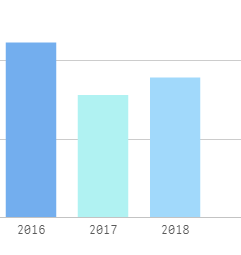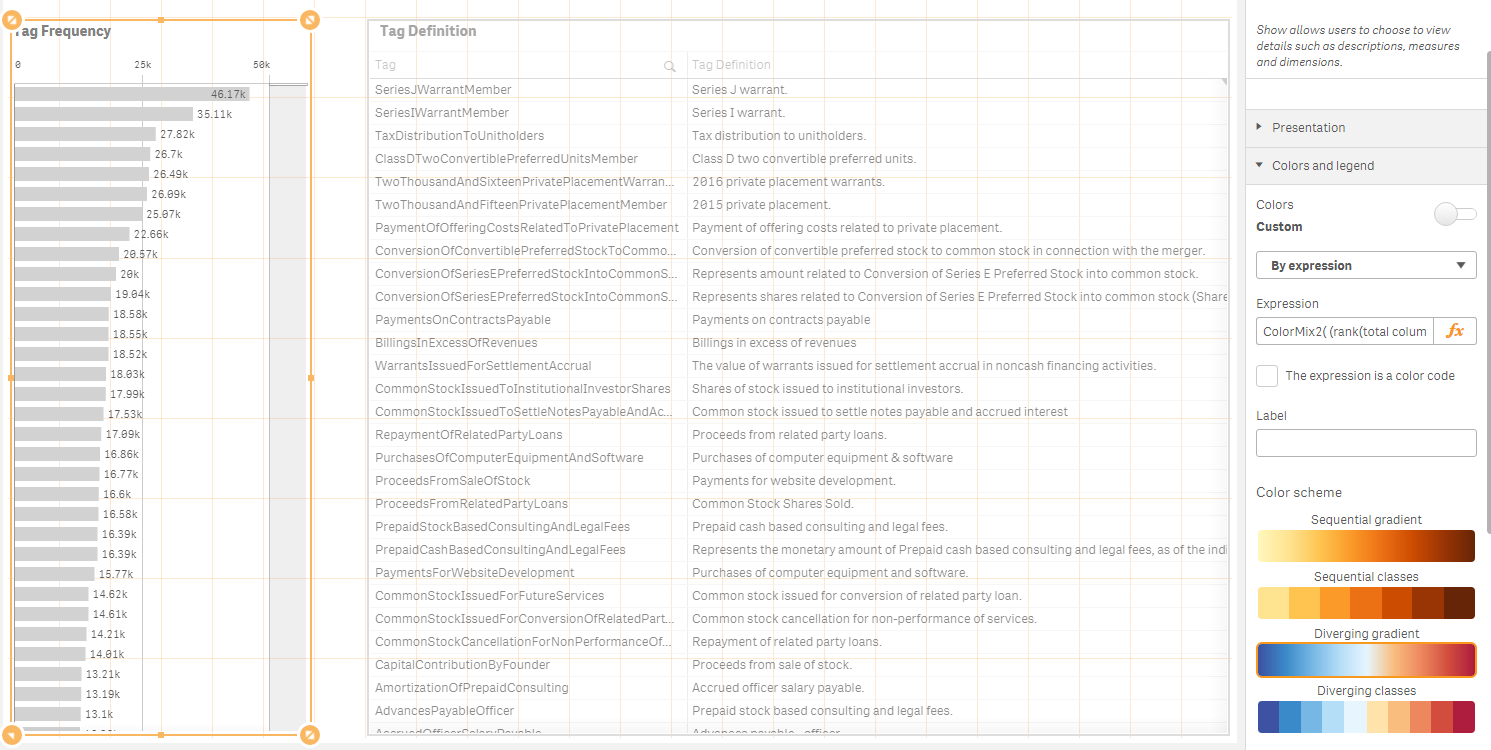# New to Qlik Sense

Discussion board where members can get started with Qlik Sense.

Highlighted
New Contributor II

## How to use colormix2 function

Hello I want to use colormix2 but I do not know how: Specifically I want to combine:

vColorLight=rgb(176,242,242)

vColorMiddle=rgb(153,204,255)

vColorMixDark=rgb(40,115,204)

in

ColorMix2( (rank(total column(1))/(noofrows(TOTAL)/2))-1 ,\$(vColorDark), \$(VColorLight),\$(vColorMiddle))

but it does not work.

I use color as expression and as color code. What am I missing?

Tags (1)
1 Solution

Accepted Solutions
Contributor II

## Re: How to use colormix2 function

Thanks for the screenshot.

Technically what you do likes fine to me....

To debug that, I would do following steps.

1. To check if the variables are working fine.

1.1 Please replace the expression with below and see if it works now.

=ColorMix2((rank(total COlumn(1))/(noofrows(TOTAL)/2))-1,rgb(153,204,255), rgb(176,242,242),rgb(40,115,204))

If works,the  issue is on your variable definitions. Please add below code in your load script.

LEt vColorLight=rgb(176,242,242);

LEt vColorMiddle=rgb(153,204,255);

LEt vColorMixDark=rgb(40,115,204);

2. If still does not work, Replace the dimension with simple one.

Your dimension looks bit complicated. It could be the issue.

3. If it still does not work, Supply me your document.

9 Replies
Contributor II

## Re: How to use colormix2 function

use below

ColorMix2( (rank(total column(1))/(noofrows(TOTAL)/2))-1 ,\$(vColorMixDark), \$(vColorLight),\$(vColorMiddle))

New Contributor II

## Re: How to use colormix2 function

it does not work.

Contributor II

## Re: How to use colormix2 function

Please find my sample as attachment.Please follow below.

1. In the load script, Add below codes

LEt vColorLight=rgb(176,242,242);

LEt vColorMiddle=rgb(153,204,255);

LEt vColorMixDark=rgb(40,115,204);

2. Create a var chart object.

3. Go to  Appearance  > Colors > Disable the auto > Choose "By Expression" > Add below expression.

ColorMix2((rank(total Column(1))/(noofrows(TOTAL)/2))-1,\$(vColorMixDark), \$(vColorLight),\$(vColorMiddle))

New Contributor II

## Re: How to use colormix2 functionNew Contributor II

## Re: How to use colormix2 functionNew Contributor II

## Re: How to use colormix2 functionContributor II

## Re: How to use colormix2 function

Thanks for the screenshot.

Technically what you do likes fine to me....

To debug that, I would do following steps.

1. To check if the variables are working fine.

1.1 Please replace the expression with below and see if it works now.

=ColorMix2((rank(total COlumn(1))/(noofrows(TOTAL)/2))-1,rgb(153,204,255), rgb(176,242,242),rgb(40,115,204))

If works,the  issue is on your variable definitions. Please add below code in your load script.

LEt vColorLight=rgb(176,242,242);

LEt vColorMiddle=rgb(153,204,255);

LEt vColorMixDark=rgb(40,115,204);

2. If still does not work, Replace the dimension with simple one.

Your dimension looks bit complicated. It could be the issue.

3. If it still does not work, Supply me your document.

New Contributor II

## Re: How to use colormix2 function

great! it wanted =ColorMix2((rank(total COlumn(1))/(noofrows(TOTAL)/2))-1,rgb(153,204,255), rgb(176,242,242),rgb(40,115,204))

Contributor II

## Re: How to use colormix2 function

Great to know!